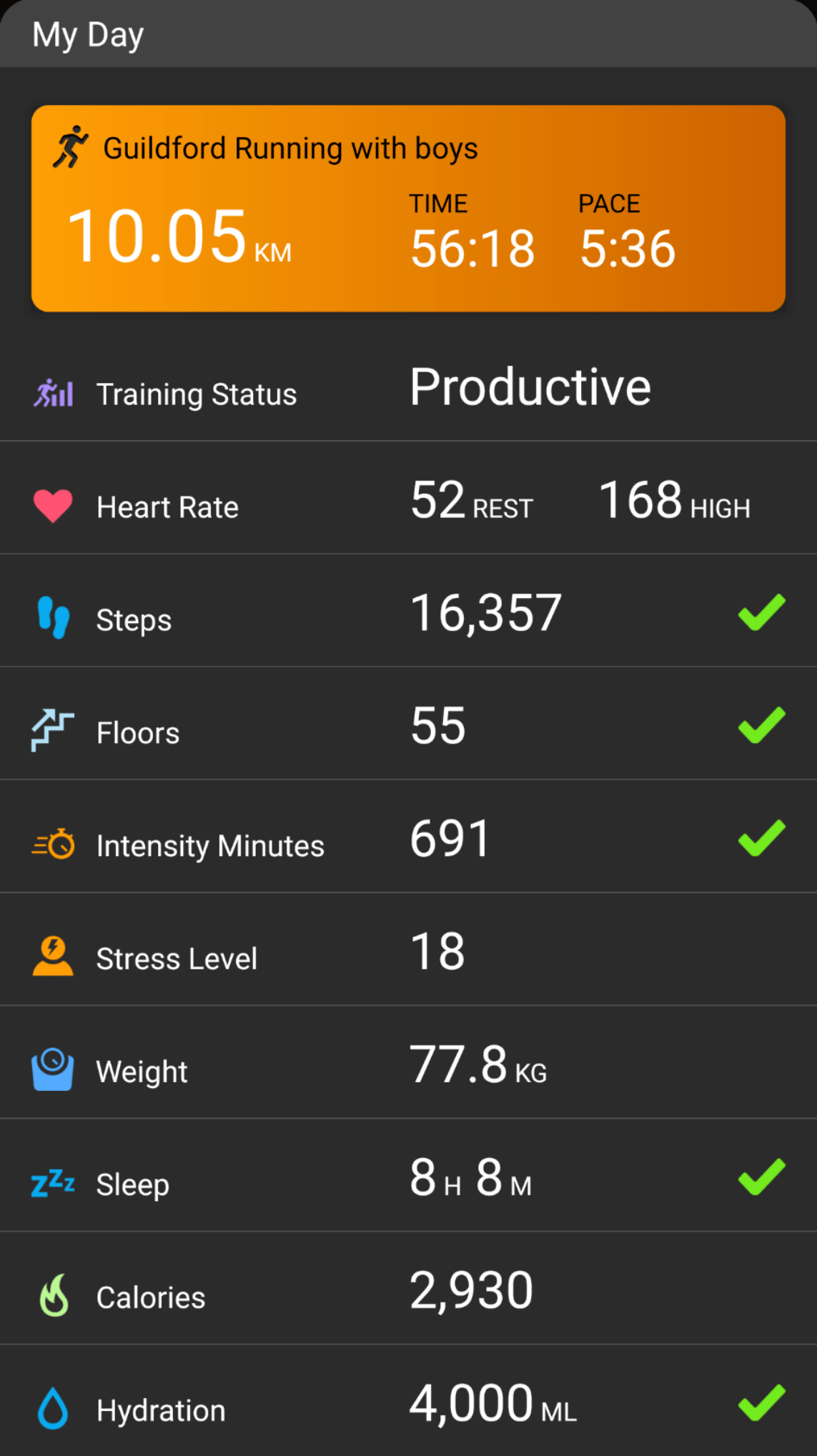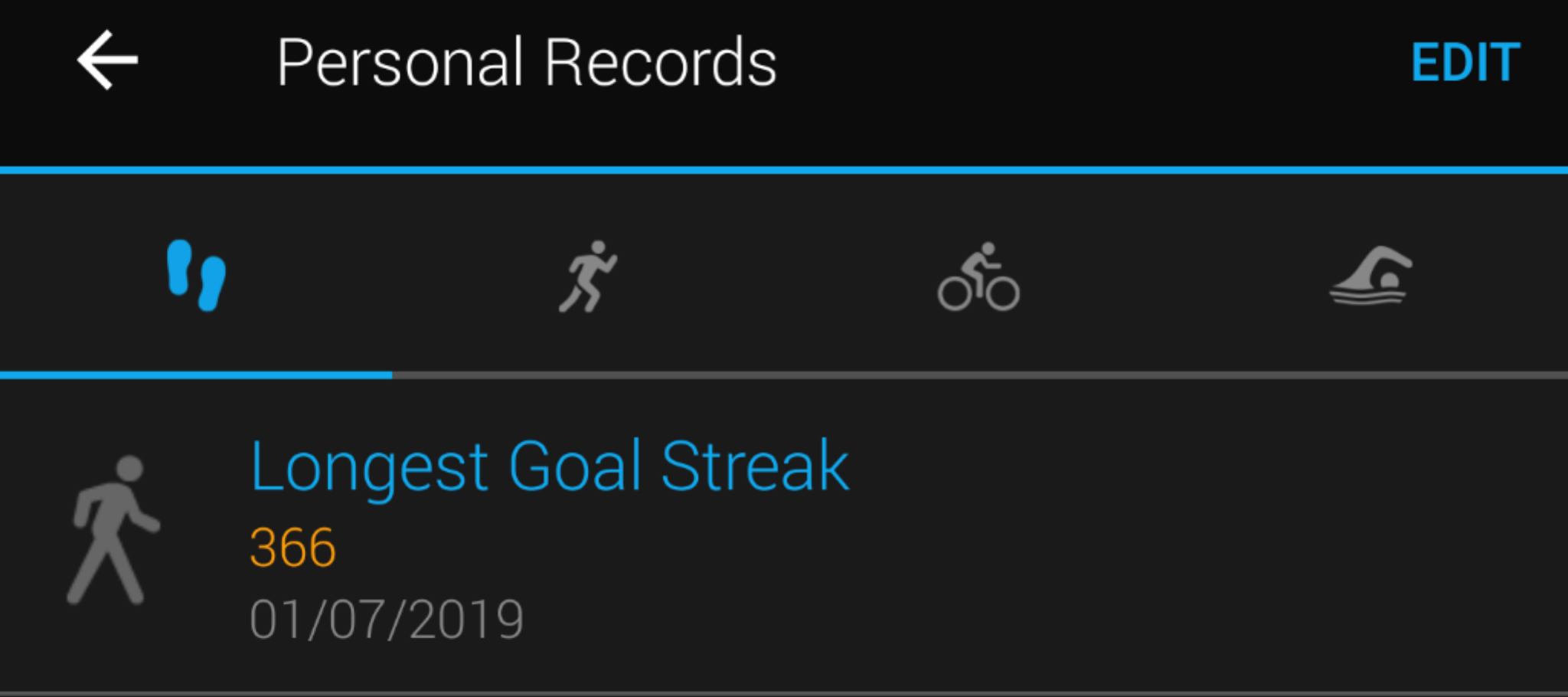## Five checkmark day

The step target is 10,000 a day. I hit it once before noon, with my run and the boys’ walk. Then I hit it again working in the garden all afternoon and evening.

I’m knackered.

## Five checkmark day

The lower activity minutes target (210 minutes vs 500 minutes earlier) may be one reason.

Mentally & emotionally disengaging at work may be the other.

## 5 check mark dayLast one was more than two months ago; I was more than two kilos lighter and much fitter.

Hopefully I won’t wait another 2+ months for another.

## Five check-mark dayA bit more discipline, and I’ll get another check mark in a few months. (Weight target: 75 Kg)

## Maths for fun on run

Doing simple maths is one of my favourite things to do on solo runs1. I try to calculate my pace based on distance and time on the watch2, or I try to calculate the time it’d take me to the finish, or most commonly, I calculate the pace I need to run to finish a race in under a certain time.

Today’s schedule had an easy 55 min run, so distance and pace weren’t very relevant. I was instead thinking whether I’ll complete 15000 steps by the time I got home.

I had about 4500 steps after the boys’ walk. I walked around the house a bit—changing, procrastinating, feeding the boy, warming up, etc. So, I could assume I would have had ~5000 steps when I started the run. Here’s a simplified version of how the calculation went:

My metronome was set at 182 beats per minute, so I could assume I’d have an average cadence of at least 182 steps per minute. Total steps would thus be…

= 182 * 55
= (180 + 2) * 55
= 180 * 55 + 2 * 55
= 180 * 55 + 110
= 180 * (1.1 * 50) + 110
= (180 * 1.1) * 50 + 110
= 198 * 50 + 110
= 198 * (100 / 2) + 110
= (198 / 2) * 100 + 110
= 99 * 100 + 110
= 9900 + 110
= 10010 steps

That should get me past the 15K mark :)

Later, on the way back up the hill, the watch buzzed to tell me I’d completed 10000 steps (for the day). I’d run ~27 mins at that point. It was the cue for the next mental maths for fun. Here’s how it went (again, grossly simplified):

Starting with total run time of 55 mins, steps I expected to get in the remainder of the run were:

= (55 – 27) * 182
= 28 * 182
= 28 * (180 + 2)
= 28 * 180 + 28 * 2
= 28 * 180 + 56
= (30 – 2) * 180 +56
= 30 * 180 – 2 * 180 +56
= 30 * 180 – 360 + 56
= 30 * 180 – 304
= 3 * 18 * 100 – 304
= 54 * 100 – 304
= 5400 – 304
= 5096 steps

Still on track to make it to 15000 steps.

Here’s what the watch said when I got home :)## Into year two…Step target streak completed a year yesterday.

## 2018 – Some successes

There were lots of setbacks in 2018 – some of them enough to drive me back into depression just by themselves. So I am not going to pore over them. Instead I am going to take note of some of the successes…

### I climbed at least 10 floors every day++I averaged about 23 floors/day, with a peak of 154 floor equivalents* on a day when I went for a long hilly run.

I climbed 10 floors even in the days after injuring my ankle. Neighbours noticed as I hobbled up and down (down was worse) our steep drive way to get those 10 floors.

### Steps target completed every day for 6 months++I finished my step target every day for the later 6 months of the year. I started on 1st July, half way through the year, and a month and a half after the injury so the foot could manage 5K+ steps without discomfort.